# Choice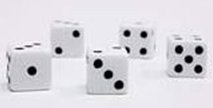by Sid Sackson

Choice is a dice game that seems quite simple at first, but it soon becomes apparent that many tricky decisions are required, hence the name. Any number can play.

1. Each turn the GM rolls 5 D6 to generate 5 numbers, e.g. 1,3,3,4,5.

2. Each player will choose one of the numbers as a "Wurfel" putting it into his Wurfel Storage area. The other four dice are used to make two numbers between 2 and 12 (two dice being used to make each number). So in the example above the player could choose 3 as his Wurfel and and make 1 + 5 = 6 and 3 + 4 = 7 as his numbers.

3. A player may store 3 different values of Wurfel in his storage area, and a player's participation in the game ends when he has 8 Wurfels of the same number in his storage area. It may be that a player cannot choose a Wurfel on a given turn as none of the numbers rolled match any of the 3 numbers he has already used for his Wurfels. In this case he simply discards one die and uses the remaining four to make 2 numbers.

4. When a player's participation ends (i.e. they have 8 Wurfels of the same value) their score is calculated as follows:

Each scoring number (2-12) will have been selected a certain number of times by the player.

• If a number has never been chosen, score 0 points.
• If a number has been chosen 1-4 times, score -200 points.
• If a number has been chosen 5 times, score 0 points.
• If a number has been chosen 6-10 times, it scores (no. of times - 5) * multiplier.
• The multipliers are 2 = 100, 3 = 70, 4 = 60, 5 = 50, 6 = 40, 7 = 30, 8 = 40, 9 = 50, 10 = 60, 11 = 70, 12 = 100.
• Eleven or more occurrences score the same as 10.

E.g. 7 4s scores 2 x 40, 9 7s scores 4 x 30 and 12 9s scores 5 x 50.

5. The game is usually played 2 turns at a time - i.e. in each postal round 2 rolls are given at the same time. Another option is to reduce the number of turns per postal round as the game progresses, e.g. 4, 4, 4, 3, 3, 3, 2, 2, 2, and then 1 thereafter.

### Suppenkasper Variant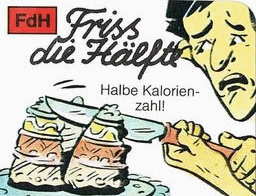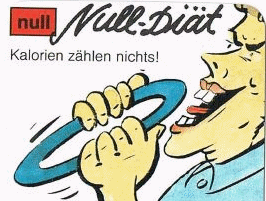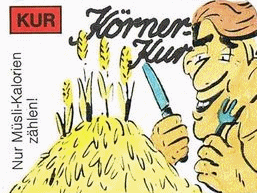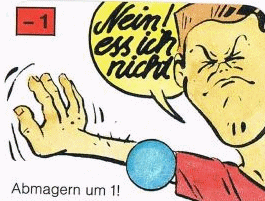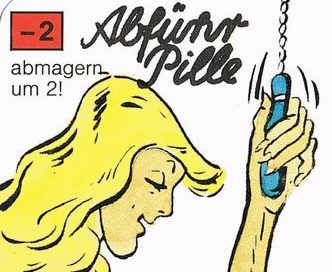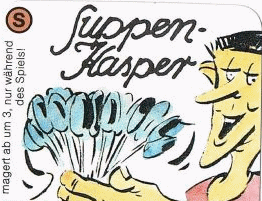As per the standard game, except that only 7 Wurfels are allowed, and each player has 6 special cards that can be played once per game:

• FdH: Remove any two numbers from the five, leaving one pair plus 1 possible wurfel
• Null: Do not take a Wurfel this round
• KUR: Take a Wurfel but no numbers this round
• -1: Refuse all the numbers this round
• -2: Refuse all the numbers and decrement the count of one of your wurfels
• Suppen-Kasper: Create an extra round from your leftovers plate

Leftovers Plate:
Any numbers not used as the result of special cards go on the player's leftovers plate. The contents of each plate are given in the turn report.

Limitations:
Only one special card can be played per round. The Suppen-Kasper card creates an extra round in which it is the special used, so none of the other special cards can be played on that round.

Suppen-Kasper:
When a player plays the Suppen-Kasper card, the fussy eater has been ordered by their mum to eat up what's on the plate. The player must select 5 numbers to eat, the rest being fed to the dog under the table. So there must be at least 5 numbers on the leftovers plate for the card to be used. The 5 chosen numbers are processed exactly as per a normal round, the only difference being the player chooses when it happens. It can be placed before or after any of the rounds in the new postal turn. So if rounds 21 and 22 are next, the extra round can be 20.5, 21.5 or 22.5.

by Richard Smith 2021 (updated 2023)